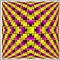# Least Squares Moving Average Color Coding[Deleted]

When I compare the MT4 Least Squares Moving Average to Tradestation's Linear Regression Curve, they are certainly the same formula. However, the MT4 indicator has some nice red/green/yellow color coding which I like a lot.
My Tradestation indicator is just one color. If you look at the MT4 Least Squares MA, the color coding logic is not simple up/down logic (ie, if MA >= MA then green, if MA < MA then red), it is something else. I like this particular indicator coloring and would like to apply it to the Tradestation Linear Regression Curve so I can use it on some non-forex markets I also trade. I am reasonably fluent in Tradestation indicator programming, but I cannot read MQL4 code at all. I'm sure I could program the color into the TS LRC indicator if I could understand the coloring logic in the MQL4 code.

So, my question is, what is the indicator color coding logic (in usual logic statements or sentences) contained in the following code?

Many Thanks, Scott

```//+------------------------------------------------------------------+
//|                                                        |
//|                                       http://www.metaquotes.net/ |
//+------------------------------------------------------------------+

//---- indicator settings
#property  indicator_chart_window
#property  indicator_buffers 3
#property indicator_color1 Yellow
#property indicator_color2 Green
#property indicator_color3 Red

//---- buffers
double ExtMapBuffer1[];
double ExtMapBuffer2[];
double ExtMapBuffer3[];
int width;

extern int Rperiod = 34;
extern int Draw4HowLongg = 1500;
int Draw4HowLong;
int shift;
int i;
int loopbegin;
double sum[];
int length;
double lengthvar;
double tmp ;
double wt[];
int c;

//+------------------------------------------------------------------+
//| Custom indicator initialization function                         |
//+------------------------------------------------------------------+
int init()
{
//---- 2 additional buffers are used for counting.
IndicatorBuffers(5);

//---- drawing settings
SetIndexBuffer(2,ExtMapBuffer1);
SetIndexBuffer(1,ExtMapBuffer2);
SetIndexBuffer(0,ExtMapBuffer3);
SetIndexBuffer(3,sum);
SetIndexBuffer(4,wt);

SetIndexStyle(2,DRAW_LINE,STYLE_SOLID,2);
SetIndexStyle(1,DRAW_LINE,STYLE_SOLID,2);
SetIndexStyle(0,DRAW_LINE,STYLE_SOLID,2);

//---- initialization done
return(0);
}

int start()

{   Draw4HowLong = Bars-Rperiod - 5;
length = Rperiod;
loopbegin = Draw4HowLong - length - 1;

for(shift = loopbegin; shift >= 0; shift--)
{
sum = 0;
for(i = length; i >= 1  ; i--)
{
lengthvar = length + 1;
lengthvar /= 3;
tmp = 0;
tmp = ( i - lengthvar)*Close[length-i+shift];
sum+=tmp;
}
wt[shift] = sum*6/(length*(length+1));

//========== COLOR CODING ===========================================

ExtMapBuffer3[shift] = wt[shift]; //red
ExtMapBuffer2[shift] = wt[shift]; //green
ExtMapBuffer1[shift] = wt[shift]; //yellow

//  for(c=loopbegin;c==shift;c++)
// {
if (wt[shift+1] > wt[shift])
{
ExtMapBuffer2[shift+1] = EMPTY_VALUE;
// ObjectCreate("smiley_face", OBJ_ARROW, 0, Time[shift], Low[shift]-Point*20);
// Print("time=  ",Time[shift]);
// ObjectSet("smiley_face", OBJPROP_ARROWCODE, 242);
// ObjectSet("smiley_face", OBJPROP_COLOR , Red);
// ObjectSet("smiley_face", OBJPROP_WIDTH  , 1);
// ObjectsRedraw();

//ExtMapBuffer3[shift+1] = EMPTY_VALUE;
//ExtMapBuffer3[shift+1] = EMPTY_VALUE;

}
else if (wt[shift+1] < wt[shift])
{
ExtMapBuffer1[shift+1] = EMPTY_VALUE; //-1 red/greem tight
//ExtMapBuffer3[shift+1] = EMPTY_VALUE;

}
else
{

ExtMapBuffer1[shift+1]=CLR_NONE;//EMPTY_VALUE;
ExtMapBuffer2[shift+1]=CLR_NONE;//EMPTY_VALUE;
}

}

return(0);
}
//+------------------------------------------------------------------+

```[Deleted]

Any takers? I'd love a little help here....Just a simple explanation of the color coding logic would make me very happy.

Best,

Scott2646

• ne color per indicator index line

when it is going up, it draws with index 2
When flat, index 1
When going down, index 3

It puts empty value into the indexes that are not used at any particular bar.1863

All I know is, in MT4 CI, the rule is : one line one color, so if there's 3 or 5 or 7 color, there's should be 3 or 5 or 7 line (and its CI buffers).

Say you want one line with 2 color, red and blue. There, you need 2 line, say the line is up, then you use the blue line and empty value the red, and vice versa.